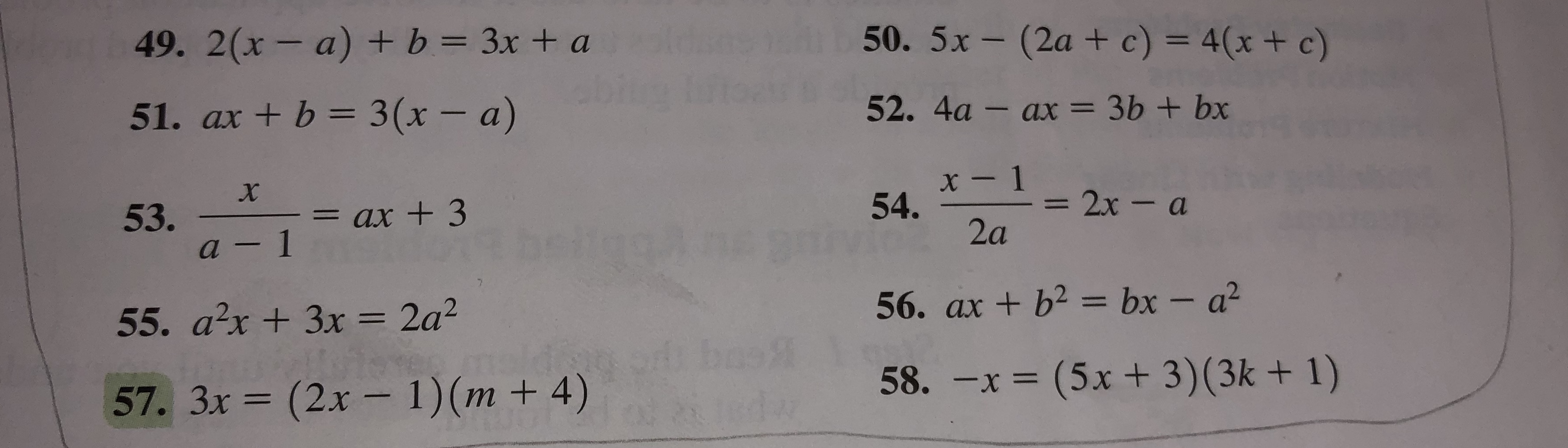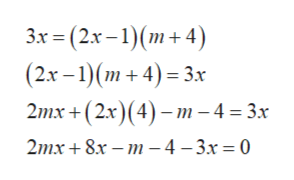50. 5x-(2a + c) = 4(x + c)49. 2(x- a) + b = 3x + a52. 4a - ax = 3b + bx51. ax + b 3 (x - a)x- 154.2x-a53.a-1= ax +32a56. ax +b2 bx - a255. a2x+3x = 2a258. -x = (5x+ 3)(3k + 1)(2x- 1)(m + 4)57. 3x(3

Question

I need assistance in solving question 57.help_outlineImage Transcriptionclose50. 5x-(2a + c) = 4(x + c) 49. 2(x- a) + b = 3x + a 52. 4a - ax = 3b + bx 51. ax + b 3 (x - a) x- 1 54. 2x-a 53. a-1 = ax +3 2a 56. ax +b2 bx - a2 55. a2x+3x = 2a2 58. -x = (5x+ 3)(3k + 1) (2x- 1)(m + 4) 57. 3x (3 fullscreen
Step 1

Solve the equation ...help_outlineImage TranscriptioncloseЗк 3 (2x -1) (m+4) (2x -1) (т + 4) - 3* 2mx + (2х)(4) — т - 4 3 3x 2mx + 8х — т - 4- 3х %3D 0 fullscreen

Want to see the full answer?

See Solution

Want to see this answer and more?

Our solutions are written by experts, many with advanced degrees, and available 24/7

See Solution
Tagged in

Equations and In-equations